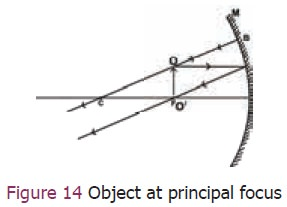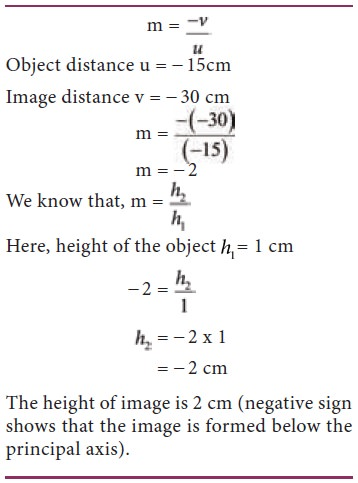Home | | Science 9th std | Concave Mirror

# Concave Mirror

We shall now find the position, size and nature of image by drawing the ray diagram for a small linear object placed on the principal axis of a concave mirror at different positions.

Concave Mirror

## 1. Ray diagrams for the formation of images

We shall now find the position, size and nature of image by drawing the ray diagram for a small linear object placed on the principal axis of a concave mirror at different positions.

Case–I: When the object is far away (at in nity), the rays of light reaching the concave mirror are parallel to each other (Figure 10).Position of the Image: e image is at the principal focus F.

Nature of the Image: It is (i) real, (ii) inverted and (iii) highly diminished in size.

Case–II: When the object is beyond the centre of curvature (Figure 11).Position of the image: Between the principal focus F and centre of curvature C.

Nature of the image: Real, inverted and smaller than object.

Case – III: When the object is at the centre of curvature (Figure 12).Position of the image: The image is at the centre of curvature itself.

Nature of the image: It is (i) Real, inverted and iii) same size as the object

Case – IV: When the object is in between the centre of curvature C and principal focus F (Figure 13).Position of the image: The image is beyond C

Nature of the image:  It  is  i)Real  ii) inverted and iii) magnified.

Case – V: When the object is at the principal focus F (Figure 14).Position of the image: Theoretically, the image is at infinity.

Nature of the image: No image can be captured on a screen nor any virtual image can be seen.

Case – VI: When the object is in between the focus F and the pole P (Figure 15).Position of the image: e image is behind the mirror.

Nature of the image: It is virtual,erect and magnified.## 2. Sign convention for measurement of distances

We follow a set of sign conventions called the cartesian sign convention. In this convention the pole (P) of the mirror is taken as the origin. The principal axis is taken as the x axis of the coordinate system (Figure 16).·        The object is always placed on the left side of the mirror.

·        All distances are measured from the pole of the mirror.

·        Distances measured in the direction of incident light are taken as positive and those measured in the opposite direction are taken as negative.

·        All distances measured perpendicular to and above the principal axis are considered to be positive.

·        All distances measured perpendicular to and below the principal axis are considered to be negative.

## 3. Mirror equation

The expression relating the distance of the object u, distance of image v and focal length f of a spherical mirror is called the mirror equation. It is given as:## 4. Linear magnification (m)

Magnification produced by a spherical mirror gives the how many times the image of an object is magnified with respect to the object size.

It can be defined as the ratio of the height of the image (hi) to the height of the object (ho).The magnification can be related to object distance (u) and the image distance (v)Note: A negative sign in the value of magni cation indicates that the image is real. A positive sign in the value of magni cation indicates that the virtual image.

### Sample Problem 1

Find the size, nature and position of image formed when an object of size 1 cm is placed at a distance of 15 cm from a concave mirror of focal length 10 cm.

1. Position of image

Object distance u =     15 cm (to the left of mirror)

Image distance v =?

Focal length f = 10 cm (concave mirror)

Using mirror formulaImage distance v = 30cm (negative sign indicates that the image is on the left side of the mirror)

Position of image is 30 cm in front of the mirror

2. Nature of image: Since the image is in front of the mirror it is real and inverted.

3. Size of image: To find the size of the image, we have to calculate the magnification.The height of image is 2 cm (negative sign shows that the image is formed below the principal axis).

### Sample Problem 2

An object 2 cm high is placed at a distance of 16 cm from a concave mirror which produces a real image 3 cm high. Find the position of the image.

Calculation of position of image## 5. Uses can cave mirror

As a dentist’s head mirror: You would have seen a circular mirror attached to a band tied to the forehead of the dentist/ENT specialist. A parallel beam of light is made to fall on the concave mirror;this mirror focuses the light beam on a small area of the body (such as teeth, throat etc.).As a make-up mirror: When a concave mirror is held near the face (between the pole and principal focus of the mirror), an upright and magnified image is seen. Here, our face will be seen magnified.

Other applications: Concave mirrors are also used as reflectors in torches, head lights in vehicles and search lights to get powerful beams of light. Concave reflectors are also used in room heaters. Large concave mirrors are used in solar heaters.Study Material, Lecturing Notes, Assignment, Reference, Wiki description explanation, brief detail
9th Science : Light : Concave Mirror |